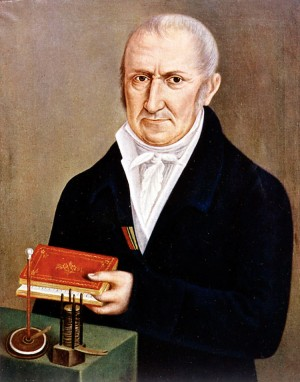# Solar Energy# Volt - Voltage UnitThe volt is the international system unit measures for measuring electromotive force and voltage.

The volt is represented by the symbol V. The measuring instrument for measuring voltage is the voltmeter.

Two different definitions of volt can be given:

On the one hand, we can consider that a volt is the voltage between two points of a conductor through which passes a current of one ampere (A) and a power of one watt (W) is dissipated.

Another alternative way to define it: The volt is equivalent to the potential difference that exists between two points of a conductor when when transporting between them a coulomb (C), the work of a July (J) is done.

The electric potential difference can be imagined as the ability to move electric charges through a resistor. In essence, the volt measures the amount of kinetic energy that each electron carries. The number of electrons is measured as electric charge, in coulombs. The volt can be multiplied by the current flow, measured in amperes (which are coulombs per second) to obtain the total electrical potential of the current in watts. The difference in electric potential was called electromotive force at a time when the word force was used in the world of physics in an uncautious way, nowadays this term is still used in some context.

The etymology of the name voltio is derived from Alessandro Volta, who in 1799 invented the voltaic battery, the first electrochemical battery. In the 1880s, the International Electricity Congress, now the International Electrotechnical Commission (IEC), approved the volt as a unit of measure for the electromotive force.

## Analogy of water flow

Sometimes, to explain the operation of an electric current, the analogy of the water flow is used. When comparing electrical circuits with water filled pipes, the voltage (difference in electrical potential) is compared to the difference in water pressure. The current is proportional to the diameter of the pipe or the amount of water that flows at that pressure. A resistor would be a reduced diameter somewhere in the pipe and a capacitor / inductor could be compared to a "U" pipe where a higher water level on one side could temporarily store energy.

The relationship between voltage and current is defined (in ohmic devices as resistances) by Ohm's law. Ohm's law is analogous to the Hagen-Poiseuille equation, since both are linear models that relate flow and potential in their respective systems.

## Definition through the Josephson effect

There has been a time when the definition of volts was given in a different way. One volt was calculated through the very precise relationship between frequency and volt with a cesium clock as a time reference. This happened with the help of a Josephson crossing. The Josephson junction consists of two superconductors that are connected by an insulator.

This relationship turned out to be independent of the disposition or materials used and, therefore, is extremely accurate. However, it is no longer the official method according to the International System of Units.

## History of the voltThis unit gets its name in honor of Alessandro Volta, who in 1800 invented the voltaic battery, the first chemical battery.

Alessandro Volta, was an Italian physicist. In the year 1800 Volta announced at the Royal Society of London the result of the experiments he had been carrying out since 1786.

With the experiments, Alessandro Volta generated electricity through different metals separated by a wet conductor. Alsessandro Volta stacked 30 metal disks separated by a cloth moistened with salt water, thus, the Italian physicist obtained electricity in the form of a continuous current.

Such a device was called "voltaic battery". From the voltaic battery arose the name with which batteries are known today and the unit of measurement of electric potential: the volt.

valoración: 3 - votos 6

Last review: April 26, 2017

Back